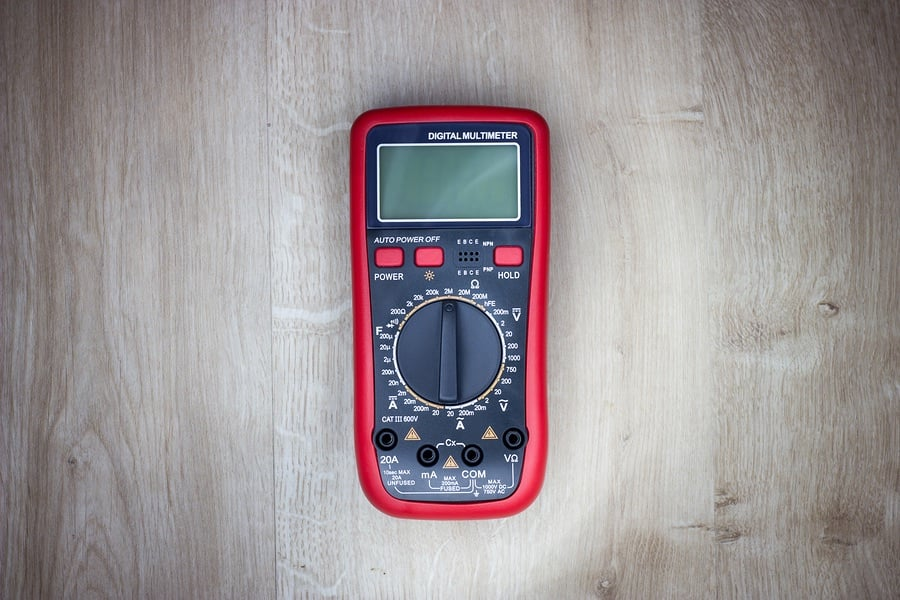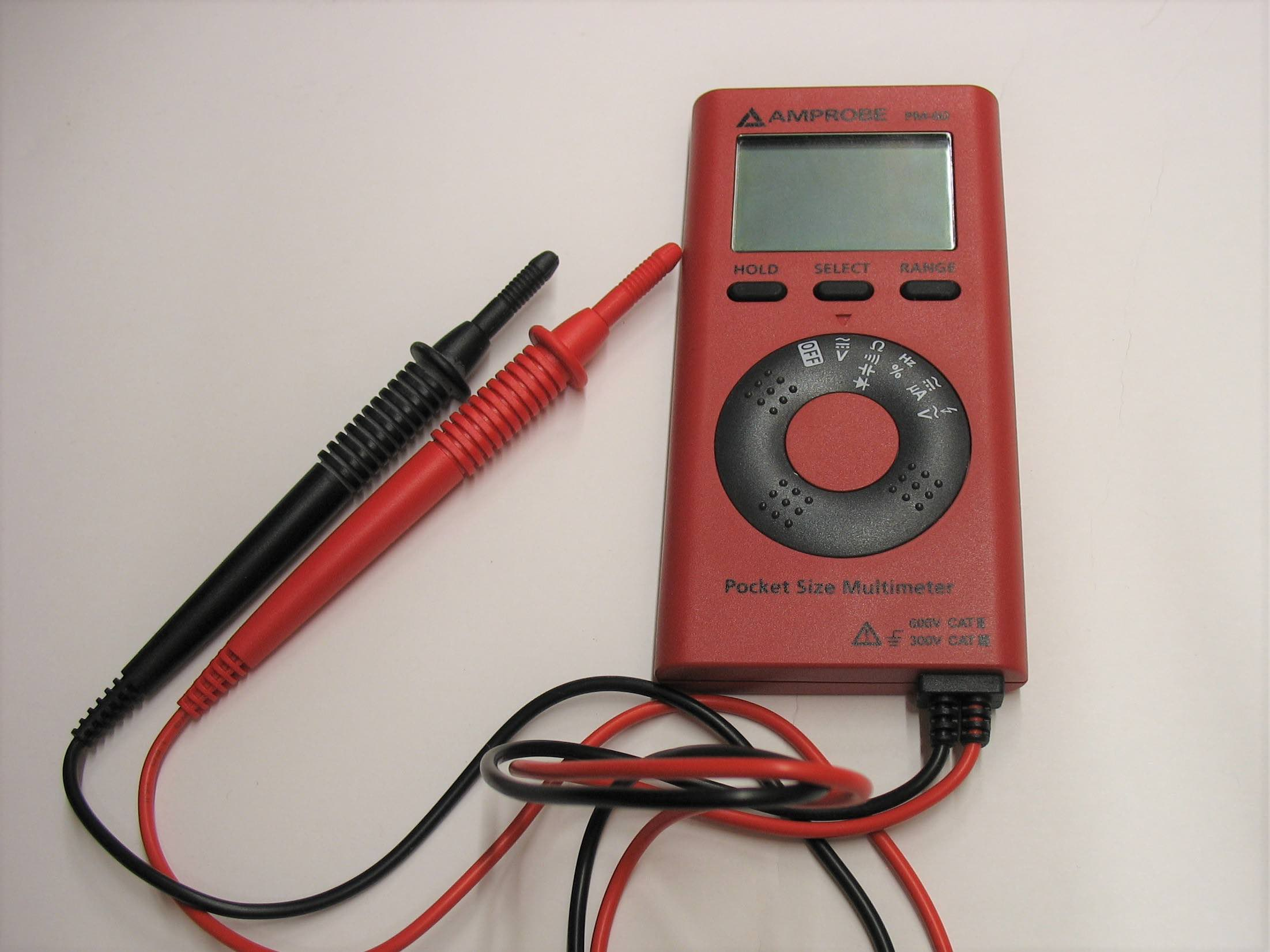Technical Article

# How to Measure AC Voltage With a Multimeter

May 19, 2020 by David Peterson

## Simple voltage measurements from a multimeter are the most frequently used function in these tools. Some of them are more difficult to understand than others, and this is definitely the case when comparing AC to DC voltage.AC voltage is important to understand because it introduces a range of other effects into the circuit which DC alone does not provide.

### How Do You Measure AC Voltage?

Measuring DC voltage with a multimeter is a simple task, place the COM lead at the reference point and the V lead at the point to be measured. The reading shows the continuous, unchanging voltage at that point. This is perfect for circuits powered by batteries and AC to DC power supplies.

In every aspect of industrial and residential electrical systems, there is another type of voltage measurement which is every bit as important, AC voltage. Simply measuring the voltage isn’t that difficult, really not much different from DC, but understanding the implications that AC has on a circuit - that is extremely important.

First, AC stands for ‘Alternating Current’ which means energy is supplied by applying a voltage in both directions, reversing the application many times per second.##### An auto range digital multimeter with LCD for measuring AC or DC voltage, current, resistance, and more.

The frequency of alternation means that it will reach a maximum voltage 60 times every second (although very briefly) then fall to a minimum voltage the same number of times, again very briefly. The voltage will spend an exactly equal amount of time positive as it does negative, which is very important to understand for voltage measurement.

### RMS or Root Mean Square

It is essential that there are two different ways to read voltage. On the DC measurement scale, you can only see the voltage as a snapshot of that exact moment. If there is any variance, it will be displayed at least as fast as the LCD screen can refresh. Trying to use this same scale to measure an alternating voltage would be too fast to see.

The voltage will change by hundreds of volts 60 times per second. The instantaneous voltage would be changing too quickly for the screen to keep up, and too fast to read even if it was capable of reading that fast.

In these alternating cases, the meter is more interested not in the instant value, but the value over a long period of time, or how much power you can actually get out of the applied voltage. This could be referred to as ‘how much DC voltage you would need to apply to get the same power output’. There are mathematical formulas that relate the minimum and maximums to the equivalent DC output.

There are a couple of strategies used in obtaining these values. Since mathematical formulas may seem like an easy solution, they are not always used because real-world situations aren’t always free of errors and anomalies. Instead, there are a few other methods:

• Use an actual DC conversion circuit to turn the AC voltage into DC and measure the output of a load resistor. Sometimes this is a small heater and the temperature is measured. This works for all kinds of unusual alternating waves.
• Convert AC to DC and getting an average, then scaling that value to the proper Root Mean Square value. Simple averages are tricky because the average of an alternating wave is zero volts! This is simple and easy, but it only works for perfect waves, which isn’t always real-world.
• Measuring and logging the voltages for a while, then calculating the Root Mean Square which has some complicated background, but boils down to individual calculations for each wave.

The first option and the last option can lead to the best results when the wave isn’t nice and perfect. This is called ‘True RMS’ and can be seen printed on most higher-quality meters.

### Other Effects from AC

Alternating voltages cause two other main changes in a circuit that must be accounted for. Therefore, it’s helpful to be able to measure how much alternating voltage has been applied, and also to use the often-included secondary frequency (Hz) measurement)

Capacitors allow through current when the wave alternates. In pure DC circuits, they have no current passing through. This current can drastically change the operating properties of a circuit if the current is flowing when it should not be. In the case of a capacitive circuit, AC current will be more than DC current.##### The ‘pocket-size’ kind of multimeter.

Inductors will provide extra resistance when an alternating voltage is applied. In a DC circuit, a coiled wire appears no different than a straight wire, but when the voltage alternates, resistance is built up. In these inductive circuits, AC current will be less than DC current.

In many cases, there will be both a DC and an AC part of the voltage. It alternates, but not perfectly to the same positive and negative levels. Some meters are able to measure this, but often an oscilloscope is required, a bit trickier than the multimeter alone.

The act of measuring AC voltage is quite simple, and identical to DC measurements. Simply place the two multimeter leads at the appropriate points in the circuit and measure the voltage difference. But it’s important to know the difference between instant values from DC and the equivalent values from AC to know which is appropriate to choose for various cases.

Like using multimeters? We do too.

So much so that we published a downloadable eBook: The Guide to Digital Multimeters

Here are the other articles in this multimeter series:•BIN95com May 22, 2020